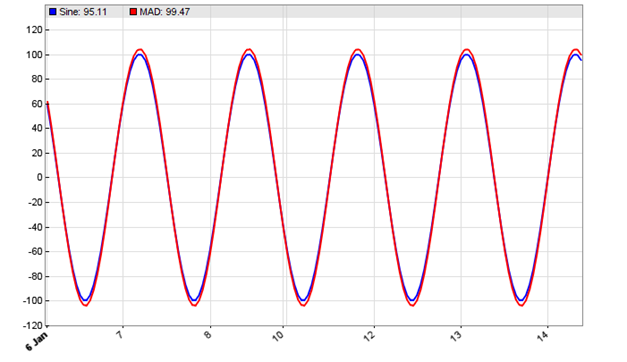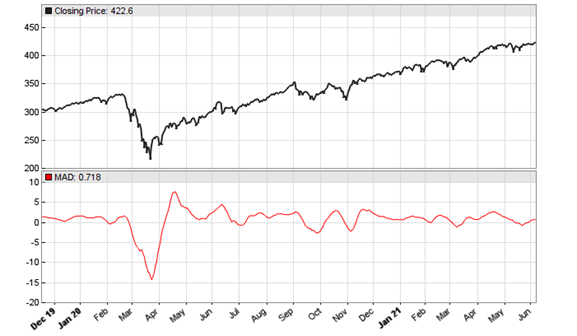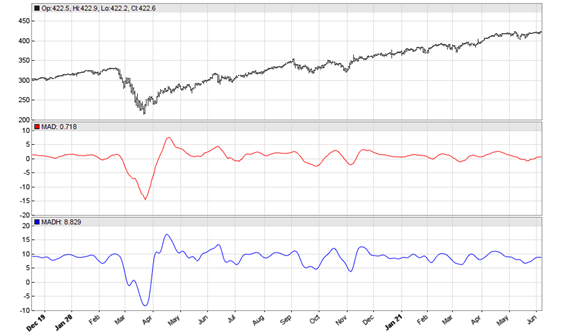As an application to the windowing technique described the the previous article, John Ehlers proposed a new trend indicator that he claimed is robust and yet simple. The latter is certainly true, as the MAD (Moving Average Difference) oscillator is, as the name says, just the difference of two moving averages normalized to +/-100.

The MAD code in C for Zorro:

```var MAD(vars Data, int ShortPeriod, int LongPeriod)
{
return 100*(SMA(Data,ShortPeriod)/SMA(Data,LongPeriod)-1.);
}
```

Why do I do a division here instead of the supposed subtraction? It saves a function call and yields the same result, because (a-b)/b = a/b – 1.

According to Ehlers, the two periods should differ by half the period of the dominant cycle in the data. This ensures that the indicator output is in phase with the dominant cycle, thus indicating its trend with no lag. For putting that to the test, we apply an SMA 5-20 difference to a 30 period sine wave (code below):

```function run()
{
MaxBars = 200;
asset(""); // dummy asset
ColorUp = ColorDn = 0; // don't plot a price curve

vars Sine = series(genSine(30,30));
var Diff = SMA(Sine,5) - SMA(Sine,20);
plot("Sine",Sine-0.5,LINE,BLUE);
}```

The resulting chart:The chart displays the SMA 5-20 difference (red) applied to a 30 period sine wave (blue). We can see that the red difference is indeed exactly in sync with the blue sine wave.

Now we apply the indicator to daily SPY data:

```void run()
{
StartDate = 20191201;
EndDate = 20210701;
BarPeriod = 1440;

asset("SPY");
}
```

The resulting chart below matches Ehlers’ example in the S&C October issue. Note that his normalization by dividing through the longer SMA introduces a small phase shift. So the chart would look slightly different with a plain SMA difference.Now let’s apply Ehlers’ Hann window technique, resulting in the MADH (MAD with Hann) indicator. We just glue both functions together:

```var MADH(vars Data, int ShortPeriod, int LongPeriod)
{
return 100*(SMA(hann(Data,ShortPeriod),ShortPeriod)
/SMA(hann(Data,LongPeriod),LongPeriod)-1.);
}```

This is the script for replicating Ehler’s chart in the S&C November issue, again with daily SPY data:

```void run()
{
StartDate = 20191201;
EndDate = 20210701;
BarPeriod = 1440;

asset("SPY");
}
```

The resulting chart:According to Ehlers, the MADH valleys and peaks are excellent buy and sell indications. Let’s not just take his word for it, but put it to the test. For this we add some buy and sell code to the end of the run() function:

```vars Signals = series(MADH(seriesC(),8,23));
if(peak(Signals))
enterShort();
else if(valley(Signals))
enterLong();```

Well. When you backtest that code, you’ll find that the MADH valleys and peaks are anything but excellent trade conditions. I do not print the equity curve here since it’s too depressing. Interestingly, the same system with the original MAD indicator produced a better result, although still not good enough for trading with real money. So whatever the MADH may be good for, I can not recommended to trade on its peaks and valleys, at least not with SPY 2019-2021. Maybe it works better with different parameters or other filter conditions.

## 3 thoughts on “The MAD indicator”

1.Henry Stern says:

As is explained in “An Introduction to Digital Signal Processing for Trend Following” (https://alphaarchitect.com/2020/08/13/an-introduction-to-digital-signal-processing-for-trend-following/), taking the difference between two moving averages with different lengths, as with the Moving Average Difference (MAD) indicator, results in a bandpass filter. A bandpass filter passes frequencies within a low and high cutoff frequency band (the bandwidth) and attenuates the others. The filter has a center (also called “resonant”) frequency between the two cutoff frequencies which it passes at maximum power. The filter bandwidth and center frequency are determined by the two moving average lengths. That is, the two moving average lengths “tune” the filter to a specific bandwidth and center frequency.

If you are able to identify the “dominant frequency” of an input signal, you can determine the two moving average lengths that will produce the desired bandwidth and center frequency, as illustrated in the 30 period sine wave example. However, financial time series are generally non-stationary, meaning that the dominant frequency changes over time. As a result, a bandpass filter with fixed coefficients will generally work better in certain time periods than others when used with a particular financial time series, but it will not perform well at all times with all time series for trading purposes. The dominant frequency of the input needs to be somewhat close to the bandpass filter center frequency, otherwise the filter output will lead or lag the input (often referred to as “divergence”), as is explained in the introduction to DSP article. Adding a window function, such as the Hann window, smooths the MAD indicator output but at the cost of some additional phase delay (lag), as will any window function (some more than others). There is an unavoidable tradeoff between smoothing and phase delay with any type of filter.

Note that the Moving Average Difference (MAD) indicator is basically the same as the Moving Average Crossover (MAC) indicator, just interpreted differently. It is also similar to the Moving Average Convergence-Divergence (MACD) indicator, which uses the difference between two exponential smoothing filters with different smoothing constants instead of two moving averages. The introduction to DSP article examines these and other popular technical indicators in detail.

2.Sam says:

It seems like all of Ehlers ideas falls apart when tested objectively.
Have you found any of his DSP work / indicators that are useful in an algo trading system? I have been banging my
Head against the wall and have tried various smoothers and filters.

3.Petra Volkova says:

Ehlers old indicators are absolutely useful, especially his frequency filters. We are using them all the time.

But it is indeed not so with his recently invented indicators. I have yet to see a useful application for them.

This site uses Akismet to reduce spam. Learn how your comment data is processed.